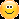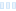Is there a way to set formula parameters programmatically, be displayed in the GUI and allow further manual tweaks?

Eg I have a formula parameter set q1,.., q8 which I give random values programmatically but then want to tweak manually.

Is there a way to set formula parameters programmatically, be displayed in the GUI and allow further manual tweaks? Eg I have a formula parameter set q1,.., q8 which I give random values programmatically but then want to tweak manually.

0

Here's an example of what I think that you want to do.

In the init section, q1 is set to a random value. The value used in the formula is tweaked by the dq1 parameter, which you can change manually.

``````example {

init:
int s = random(@seed)
complex q1 = (-0.5,-0.5) + (abs(s) + flip(random(s)))/#randomrange
q1 = q1 + @dq1

loop:
z = sqr(z + q1) + #pixel

bailout:
|z| <= @bailout

default:
title = "example"
complex param dq1
default = (0,0)
endparam
int param seed
caption = "seed"
default = 123456789
endparam
float param bailout
caption = "Bailout value"
default = 4.0
min = 1.0
endparam

}
``````
Here&#039;s an example of what I think that you want to do. In the init section, q1 is set to a random value. The value used in the formula is tweaked by the dq1 parameter, which you can change manually. ```` example { init: int s = random(@seed) complex q1 = (-0.5,-0.5) + (abs(s) + flip(random(s)))/#randomrange q1 = q1 + @dq1 loop: z = sqr(z + q1) + #pixel bailout: |z| &lt;= @bailout default: title = &quot;example&quot; complex param dq1 caption = &quot;add to q1&quot; default = (0,0) endparam int param seed caption = &quot;seed&quot; default = 123456789 endparam float param bailout caption = &quot;Bailout value&quot; default = 4.0 min = 1.0 endparam } ````

0

Yes that workaround is what I do now but I don't want to.

In your example I want a parameter @q1, filled with that calculated vale, and showing up in GUI for further tweaking.

My question is is there a way to programmatically do the equivalent of assignments like @q = value? No, I think, but asking anyways.

Yes that workaround is what I do now but I don&#039;t want to. In your example I want a parameter @q1, filled with that calculated vale, and showing up in GUI for further tweaking. My question is is there a way to programmatically do the equivalent of assignments like @q = value? No, I think, but asking anyways.

0

At the moment there is no way to do that. I've got a feature request about this on my list already.Ultra Fractal author

0
63
views
3
replies
3
followers
live preview
Enter at least 10 characters.
WARNING: You mentioned %MENTIONS%, but they cannot see this message and will not be notifiedSaving...
Saved
All posts under this topic will be deleted ?
Pending draft ... Click to resume editing